# albany.eduMy Questions | bartlebyForum: Week Three: Prejudice and Discrimination ( Due...Mail - Mangia, Louis M - Outlookhttps://www.albany.edu/~by872279/micro/Hw1.pdf4. Suppose a person has \$8 to spend only on apples and bananas. Apples1cost \$0.4 each, and bananas cost \$0.1 each. Furthermore, his preferences forapples (A) and bananas (B) can be represented by U = VAB.•11 albany.eduMy Questions | bartlebyMail - Mangia, Louis M - OutlookForum: Week Three: Prejudice and Discrimination ( Due ...https://www.albany.edu/~by872279/micro/Hw1.pdfcost \$0.4 each, and bananas cost \$0.1 each. Furthermore, his preferences forapples (A) and bananas (B) can be represented by U = VAB.a) If A = 5 and B = 80, what will utility be?b) If A = 10, what value of for B will provide the same utility in part a?c) If A = 20, what value of for B will provide the same utility in partsa and b?d) Graph the indifference curve implied by parts a through c.e) Give the budget constraint, which of the points identified in parts athrough c can be bought by this person?f) show through some examples thatof allocating incomeothereverywayprovides less utility than does the point identified in part b. graph this utilitymaximizing situation.

Question
2 views

4. Suppose a person has \$8 to spend only on apples and bananas. Apples

1

cost \$0:4 each, and bananas cost \$0:1 each. Furthermore, his preferences for apples (A) and bananas (B) can be represented by U = pAB.

a) If A = 5 and B = 80, what will utility be?
b) If A = 10, what value of for B will provide the same utility in part a? c) If A = 20, what value of for B will provide the same utility in parts

a and b?
d) Graph the indi§erence curve implied by parts a through c.
e) Give the budget constraint, which of the points identiÖed in parts a

through c can be bought by this person?
f) show through some examples that every other way of allocating income

provides less utility than does the point identiÖed in part b. graph this utility maximizing situation.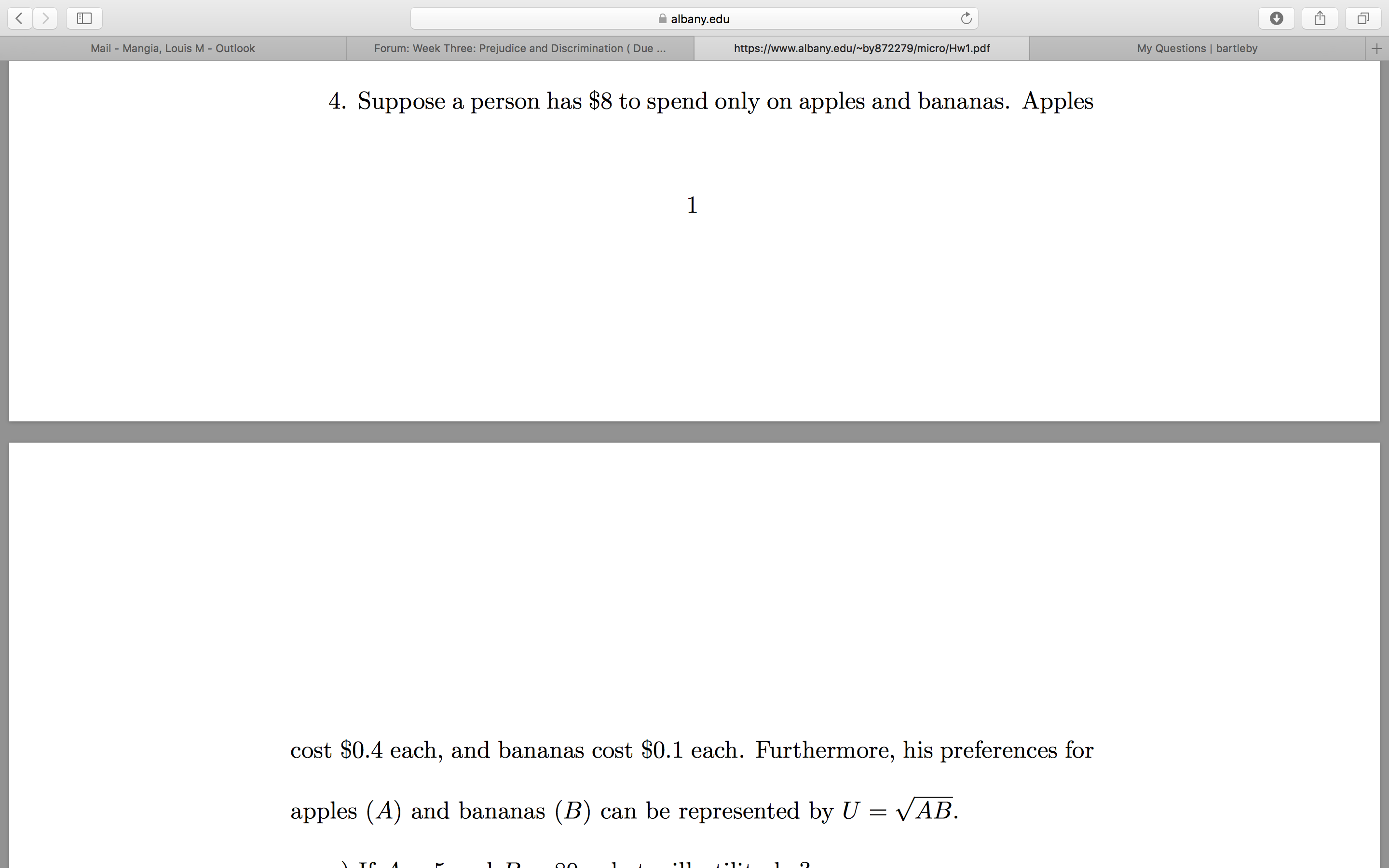help_outlineImage Transcriptionclosealbany.edu My Questions | bartleby Forum: Week Three: Prejudice and Discrimination ( Due... Mail - Mangia, Louis M - Outlook https://www.albany.edu/~by872279/micro/Hw1.pdf 4. Suppose a person has \$8 to spend only on apples and bananas. Apples 1 cost \$0.4 each, and bananas cost \$0.1 each. Furthermore, his preferences for apples (A) and bananas (B) can be represented by U = VAB. •11 fullscreen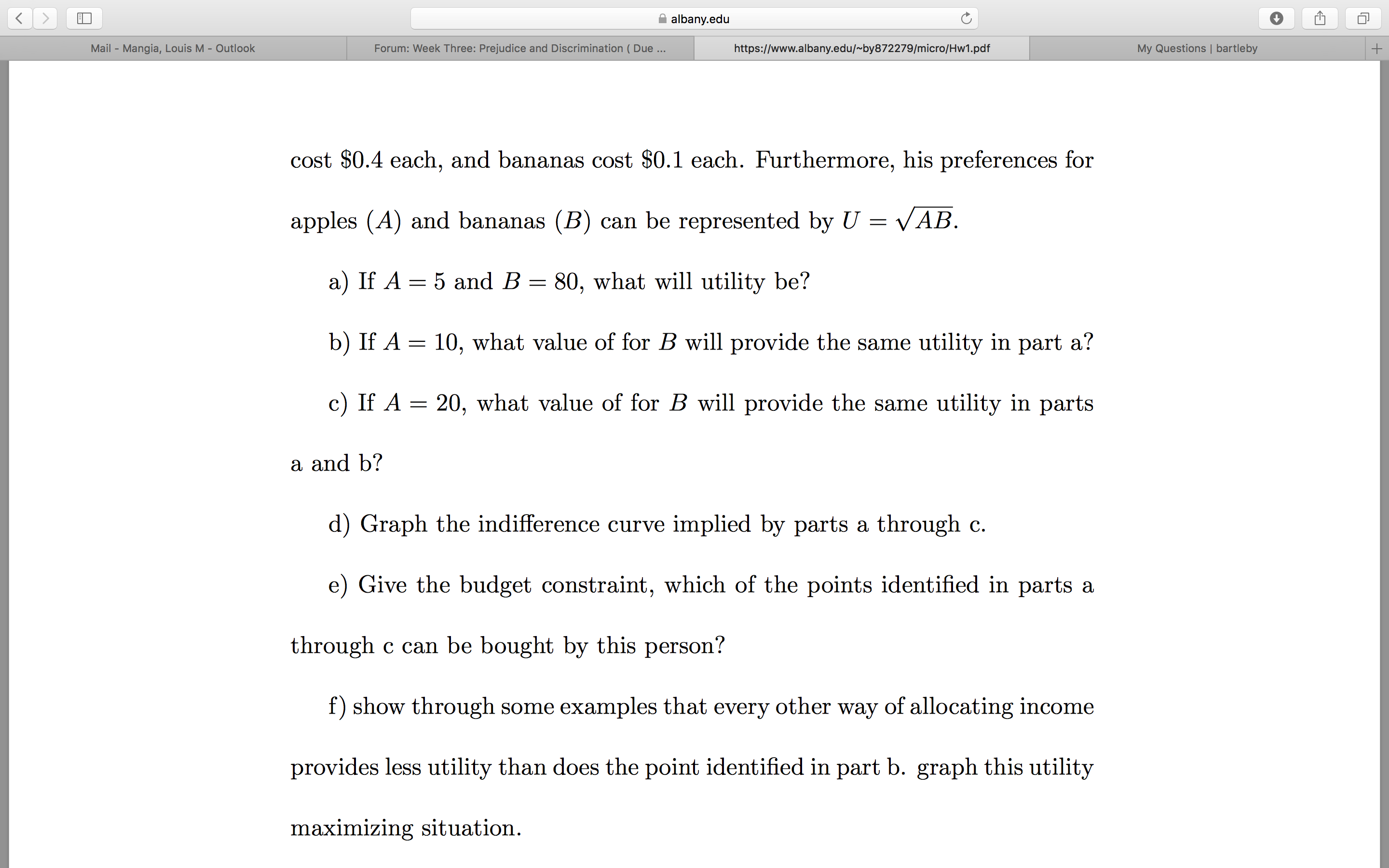help_outlineImage Transcriptionclosealbany.edu My Questions | bartleby Mail - Mangia, Louis M - Outlook Forum: Week Three: Prejudice and Discrimination ( Due ... https://www.albany.edu/~by872279/micro/Hw1.pdf cost \$0.4 each, and bananas cost \$0.1 each. Furthermore, his preferences for apples (A) and bananas (B) can be represented by U = VAB. a) If A = 5 and B = 80, what will utility be? b) If A = 10, what value of for B will provide the same utility in part a? c) If A = 20, what value of for B will provide the same utility in parts a and b? d) Graph the indifference curve implied by parts a through c. e) Give the budget constraint, which of the points identified in parts a through c can be bought by this person? f) show through some examples that of allocating income other every way provides less utility than does the point identified in part b. graph this utility maximizing situation. fullscreen
check_circle

Step 1

Hey, since there are multiple subparts in the question, we will answer the first three subparts. If you want any specific subpart to be answered then please submit the question again and specify the subpart in your message.

(a)

The utility from apple and banana is given as follows.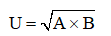Step 2

If A =5 and B = 80 we can calculate the utility as follows.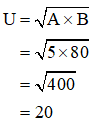Step 3

(b)

If A = 10 and U = 20 we can calculate the value for B as follows.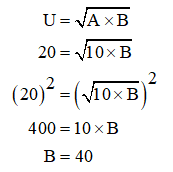...

### Want to see the full answer?

See Solution

#### Want to see this answer and more?

Solutions are written by subject experts who are available 24/7. Questions are typically answered within 1 hour.*

See Solution
*Response times may vary by subject and question.
Tagged in